# Find Circles and Ellipses in an Image using OpenCV | Python

To identify circles, ellipses or in general any shape in which the pixels are connected we use the `SimpleBlobDetector()` function of OpenCV. In non-technical terms, a blob is understood as a thick liquid drop. Here, we are going to call all shapes as a blob. Our task is to detect and recognize whether the blob is a circle or not.

OpenCV provides a convenient way to detect blobs and filter them based on different characteristics. There are various different parameters that control the identification process and the results. The important parameters used for this project are:

• Filter by Area – This is to avoid any identification of any small dots present in the image that can be wrongly detected as a circle.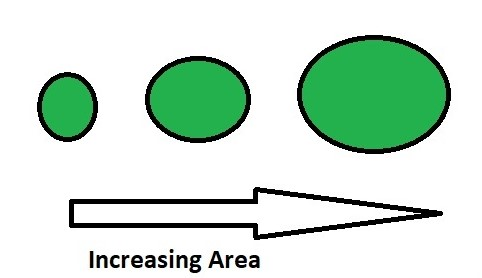• Filter by Circularity – This helps us to identify, shapes that are more similar to a circle.
`Circularity =. `

A true circle has circularity of 1, a square has a circularity near 78%.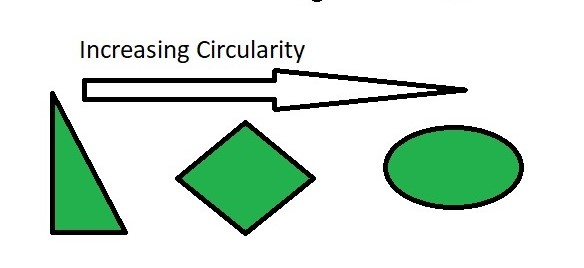• Filter by Convexity – Concavity in general, destroys the circularity.More is the convexity, the closer it is to a close circle.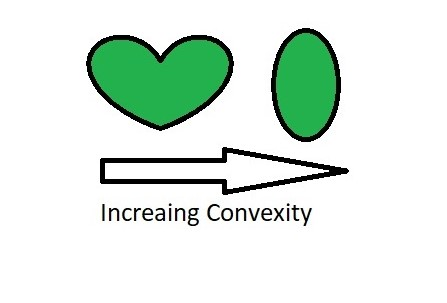• Filter by Inertia – Objecs similar to a circle has larger inertial.E.g. for a circle, this value is 1, for an ellipse it is between 0 and 1, and for a line it is 0. To filter by inertia ratio, set filterByInertia = 1, and set, 0 <= minInertiaRatio <= 1 and maxInertiaRatio (<=1 ) appropriately.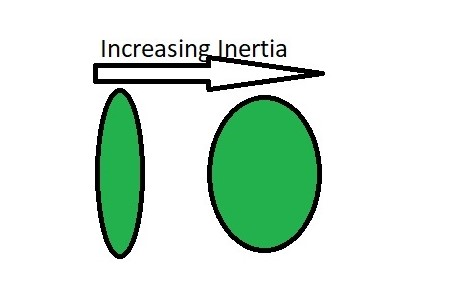Below is the code for identifying Circles:

 `import` `cv2 ` `import` `numpy as np ` ` `  `# Load image ` `image ``=` `cv2.imread(``'C://gfg//images//blobs.jpg'``, ``0``) ` ` `  `# Set our filtering parameters ` `# Initialize parameter settiing using cv2.SimpleBlobDetector ` `params ``=` `cv2.SimpleBlobDetector_Params() ` ` `  `# Set Area filtering parameters ` `params.filterByArea ``=` `True` `params.minArea ``=` `100` ` `  `# Set Circularity filtering parameters ` `params.filterByCircularity ``=` `True`  `params.minCircularity ``=` `0.9` ` `  `# Set Convexity filtering parameters ` `params.filterByConvexity ``=` `True` `params.minConvexity ``=` `0.2` `     `  `# Set inertia filtering parameters ` `params.filterByInertia ``=` `True` `params.minInertiaRatio ``=` `0.01` ` `  `# Create a detector with the parameters ` `detector ``=` `cv2.SimpleBlobDetector_create(params) ` `     `  `# Detect blobs ` `keypoints ``=` `detector.detect(image) ` ` `  `# Draw blobs on our image as red circles ` `blank ``=` `np.zeros((``1``, ``1``))  ` `blobs ``=` `cv2.drawKeypoints(image, keypoints, blank, (``0``, ``0``, ``255``), ` `                          ``cv2.DRAW_MATCHES_FLAGS_DRAW_RICH_KEYPOINTS) ` ` `  `number_of_blobs ``=` `len``(keypoints) ` `text ``=` `"Number of Circular Blobs: "` `+` `str``(``len``(keypoints)) ` `cv2.putText(blobs, text, (``20``, ``550``), ` `            ``cv2.FONT_HERSHEY_SIMPLEX, ``1``, (``0``, ``100``, ``255``), ``2``) ` ` `  `# Show blobs ` `cv2.imshow(``"Filtering Circular Blobs Only"``, blobs) ` `cv2.waitKey(``0``) ` `cv2.destroyAllWindows() `

Output: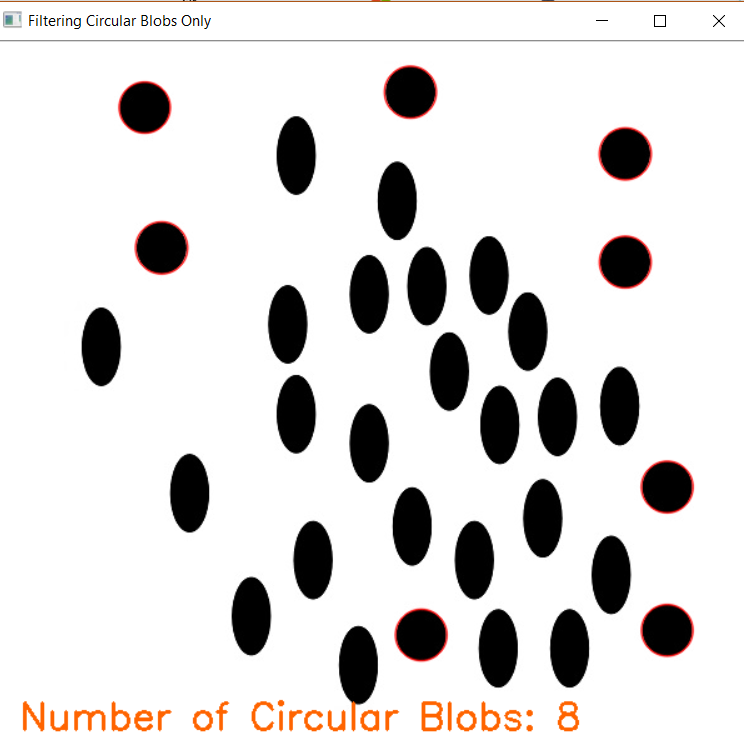Attention geek! Strengthen your foundations with the Python Programming Foundation Course and learn the basics.

To begin with, your interview preparations Enhance your Data Structures concepts with the Python DS Course.

My Personal Notes arrow_drop_up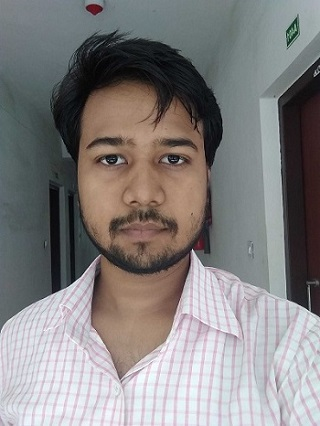Check out this Author's contributed articles.

If you like GeeksforGeeks and would like to contribute, you can also write an article using contribute.geeksforgeeks.org or mail your article to contribute@geeksforgeeks.org. See your article appearing on the GeeksforGeeks main page and help other Geeks.

Please Improve this article if you find anything incorrect by clicking on the "Improve Article" button below.

Article Tags :

1

Please write to us at contribute@geeksforgeeks.org to report any issue with the above content.